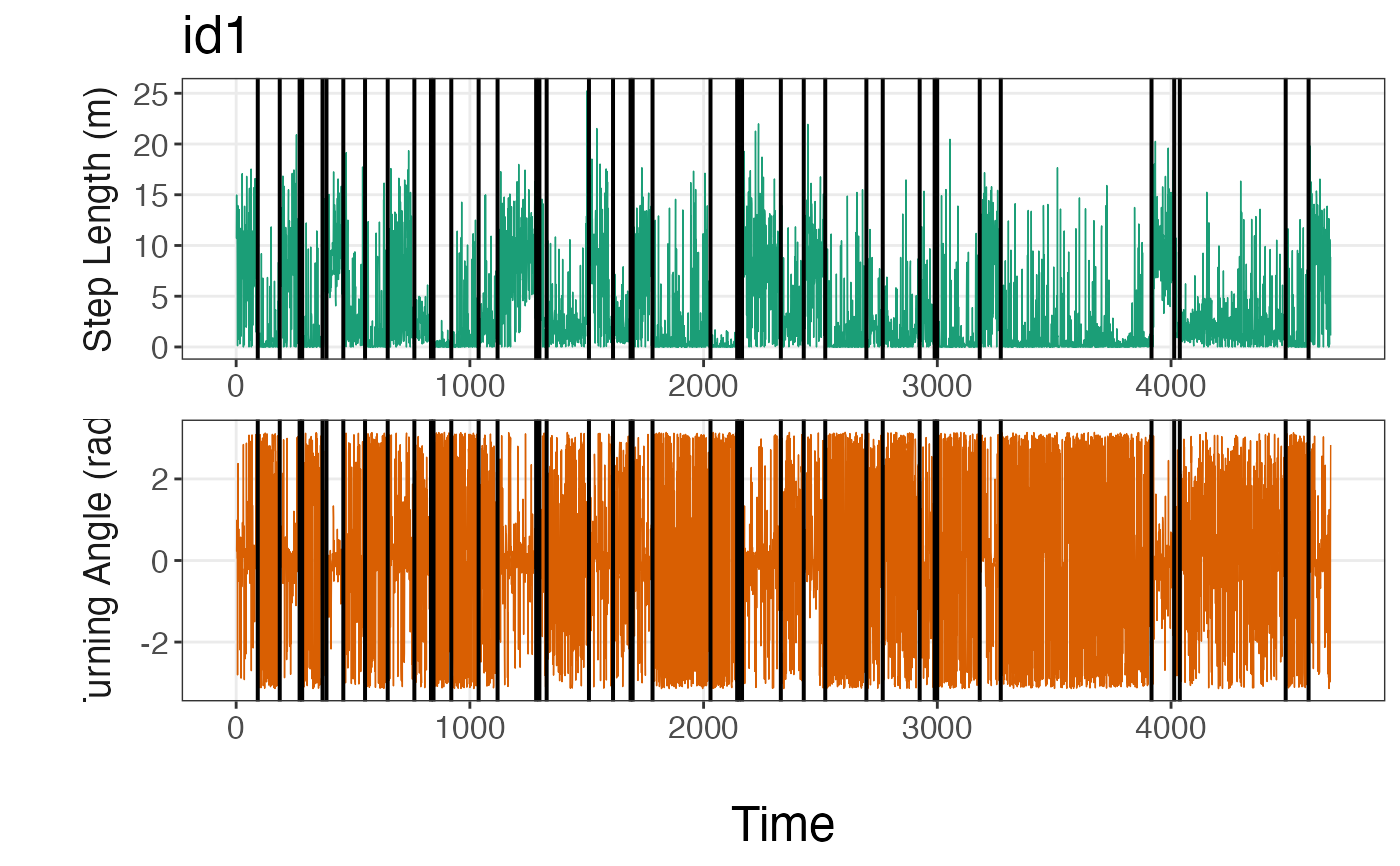Visualize the breakpoints estimated by the segmentation model as they relate to either the original (continuous) or discretized data. These plots assist in determining whether too many or too few breakpoints were estimated as well as whether the user needs to redefine how they discretized their data before analysis.

plot_breakpoints(data, as_date = FALSE, var_names, var_labels = NULL, brkpts)

## Arguments

data A list where each element stores a data frame for a given animal ID. Each of these data frames contains columns for the ID, date or time1 generated by filter_time, as well as each of the movement variables analyzed by segment_behavior. logical. If TRUE, plots breakpoints and data streams over the date. By default, this is set to FALSE. A vector of the column names for the movement variables to be plotted over time. A vector of the labels to be plotted on the y-axis for each movement variable. Set to NULL by default. A data frame that contains the breakpoints associated with each animal ID. This data frame is returned by get_breakpts.

## Value

A line plot per animal ID for each movement variable showing how the estimated breakpoints relate to the underlying data. Depending on the user input for var_names, this may either be on the scale of the original continuous data or the discretized data.

## Examples


# \donttest{
data(tracks.list)

#subset only first track
tracks.list<- tracks.list

#only retain id and discretized step length (SL) and turning angle (TA) columns
tracks.list2<- purrr::map(tracks.list,
subset,
select = c(id, SL, TA))

set.seed(1)

# Define model params
alpha<- 1
ngibbs<- 1000
nbins<- c(5,8)

#future::plan(future::multisession)  #run all MCMC chains in parallel
dat.res<- segment_behavior(data = tracks.list2, ngibbs = ngibbs, nbins = nbins,
alpha = alpha)
#> 1.353 sec elapsed

# Determine MAP iteration for selecting breakpoints and store breakpoints
MAP.est<- get_MAP(dat = dat.res$LML, nburn = ngibbs/2) brkpts<- get_breakpts(dat = dat.res$brkpts, MAP.est = MAP.est)

#run function
plot_breakpoints(data = tracks.list, as_date = FALSE, var_names = c("step","angle"),
var_labels = c("Step Length (m)", "Turning Angle (rad)"), brkpts = brkpts)
#> Warning: It is deprecated to specify guide = FALSE to remove a guide. Please use guide = "none" instead.# }